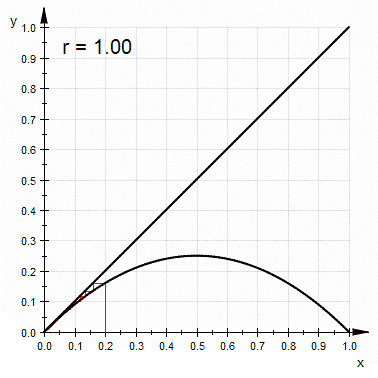# Let’s Roll a Die

Let’s say I want to create the ability to roll a die in my program. Without giving it a second thought, we would call the the built-in function Math.random() in JavaScript (or the equivalent in any other language) and tweak it with some simple manipulation to our desired range. But is this number actually random? The dictionary definition of random is described as follows:

# Pseudo-Random Number Generator

Random numbers generated by our programs are actually pseudo-random number because numbers generated by an algorithm is by definition not random. However these numbers are seemingly random enough that most users don’t complain about them. There are many implementations of pseudorandom number generator (PRNG) and they vary from language to language. Many PRNGs today use the linear congruential method. Here is a formula of how to get the next random number xₙ₊₁ based on the previous random number xₙ:

`function randomNumGenerator(k, c, m, seed, loop) {    let prevNum = seed    let randNums = [seed]    for(let i = 0; i < loop; i++) {        prevNum = (k * prevNum + c) % m        randNums.push(prevNum)    }    console.log(randNums.join())}randomNumGenerator(23, 51, 100, 19, 22)//=>19,88,75,76,99,28,95,36,79,68,15,96,59,8,35,56,39,48,55,16,19,88`

# Can I Be More ‘Random’?

We already expected the numbers to repeat since we gave it a formula to generate the numbers. The question we should be asking is not whether or not they will repeat but rather how long until they repeat.

`randomNumGenerator(23, 51, 101, 19, 50)//=>19,84,64,8,33,2,97,60,17,38,16,15,93,69,22,52,35,48,44,53,58,72,91,23,75,59,95,14,70,45,76,82,18,61,40,62,63,86,9,56,26,43,30,34,25,20,6,88,55,3,19`

## Logistic Map

We can further improve our appearance of randomness by leveraging logistic map that’s used to illustrate chaos in simple non-linear dynamic systems.A cobweb diagram of the logistic map, showing chaotic behaviour for most values of r > 3.57 (Wikipedia)

## Still don’t believe in your programming language?

Your computer is actually pretty good at generating random numbers. Not the programming language, but the computer itself. There are numbers that can be created that are closer to true randomness via hardware random number generator(HRNG). These numbers are generated from physical processes instead of an algorithm. Your operating system has a much bigger source of data than a user chosen seed value to create a random number such as CPU temperature, number of seconds since last key was pressed, number of pixels from previous mouse position. These events are likely unpredictable and mirror randomness much closer.

# How Do I Know If I Look Random Enough?

To the human eye, if we do not recognize a pattern we would hastily deem something to be random. For example, let’s take a look at the below two strings.

`string1 = "abcabcabcabcabcabcabcabcabcabc"string2 = "31832jdksnckjanlml201lakcnqwli"`
`function generateString1() {    return "abc".repeat(10)}function generateString2() {    return "31832jdksnckjanlml201lakcnqwli"}`
`function generateString1() {    return "abc".repeat(20)}//=>abcabcabcabcabcabcabcabcabcabcabcabcabcabcabcabcabcabcabcabcfunction generateString2() {    return "31832jdksnckjanlml201lakgcnqwli92jsksmz01pq26hsunx81lz01me92"}//=>31832jdksnckjanlml201lakgcnqwli92jsksmz01pq26hsunx81lz01me92`